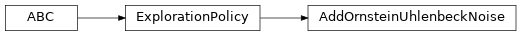# garage.np.exploration_policies.add_ornstein_uhlenbeck_noise¶

Ornstein-Uhlenbeck exploration strategy.

Ornstein-Uhlenbeck exploration strategy comes from the Ornstein-Uhlenbeck process. It is often used in DDPG algorithm because in continuous control task it is better to have temporally correlated exploration to get smoother transitions. And OU process is relatively smooth in time.

class AddOrnsteinUhlenbeckNoise(env_spec, policy, *, mu=0, sigma=0.3, theta=0.15, dt=0.01, x0=None)[source]An exploration strategy based on the Ornstein-Uhlenbeck process.

The process is governed by the following stochastic differential equation.

$dx_t = -\theta(\mu - x_t)dt + \sigma \sqrt{dt} \mathcal{N}(\mathbb{0}, \mathbb{1}) # noqa: E501$
Parameters
• env_spec (EnvSpec) – Environment to explore.

• policy (garage.Policy) – Policy to wrap.

• mu (float) – $$\mu$$ parameter of this OU process. This is the drift component.

• sigma (float) – $$\sigma > 0$$ parameter of this OU process. This is the coefficient for the Wiener process component. Must be greater than zero.

• theta (float) – $$\theta > 0$$ parameter of this OU process. Must be greater than zero.

• dt (float) – Time-step quantum $$dt > 0$$ of this OU process. Must be greater than zero.

• x0 (float) – Initial state $$x_0$$ of this OU process.

reset(self, dones=None)[source]

Reset the state of the exploration.

Parameters

dones (List[bool] or numpy.ndarray or None) – Which vectorization states to reset.

get_action(self, observation)[source]

Return an action with noise.

Parameters

observation (np.ndarray) – Observation from the environment.

Returns

An action with noise. dict: Arbitrary policy state information (agent_info).

Return type

np.ndarray

get_actions(self, observations)[source]

Return actions with noise.

Parameters

observations (np.ndarray) – Observation from the environment.

Returns

Actions with noise. List[dict]: Arbitrary policy state information (agent_info).

Return type

np.ndarray

update(self, episode_batch)

Update the exploration policy using a batch of trajectories.

Parameters

episode_batch (EpisodeBatch) – A batch of trajectories which were sampled with this policy active.

get_param_values(self)

Get parameter values.

Returns

Values of each parameter.

Return type

list or dict

set_param_values(self, params)

Set param values.

Parameters

params (np.ndarray) – A numpy array of parameter values.